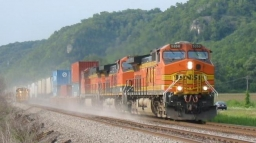# Direction 7554

A freight train departs from one station at an average speed of 36 km/h. 2 hours later, personal pressure leaves in the same direction at an average speed of 48 km/h. After how many hours will the passenger train catch up with the freight train?

t =  6 h

### Step-by-step explanation:

36·(t+2) = 48·t

12t = 72

t = 72/12 = 6

t = 6

Our simple equation calculator calculates it.Did you find an error or inaccuracy? Feel free to write us. Thank you!

Tips for related online calculators
Do you have a linear equation or system of equations and looking for its solution? Or do you have a quadratic equation?
Do you want to convert velocity (speed) units?
Do you want to convert time units like minutes to seconds?